R-suared (R2) is a statistic that explains the amount of variance accounted for in the relationship between two (or more) variables. Sometime R2 is called the coefficient of determination, and it is given as the square of a correlation coefficient.

Given paired variables (Xi, Yi), a linear model that explains the relationship between the variables is given by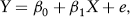where e is a mean zero error. The parameters of the linear model can be estimated using the least squares method and denoted by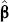and1, respectively. The parameters are estimated by minimizing the sum of squared residuals between variable Yi and the model β0 + β1Xi, that is,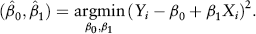It can be shown that the least squares estimations are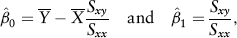where the sample cross-covariance Sxy is defined as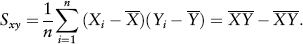Statistical packages such as ...

•••